# Grams and Kilograms

Grams and Kilograms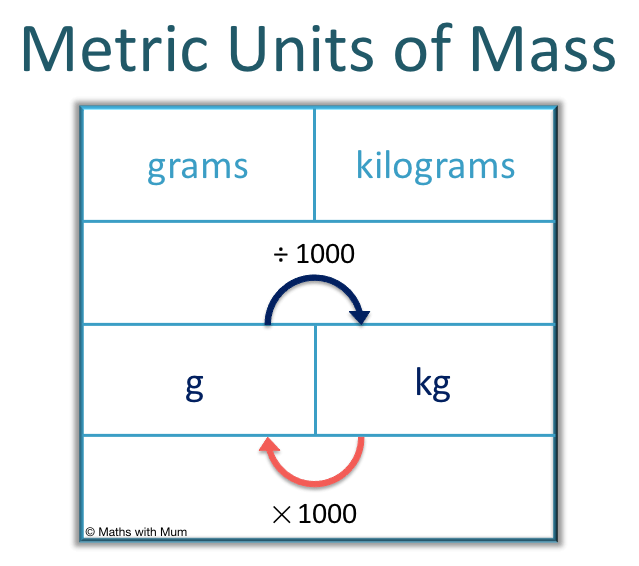• 1 gram weighs about the same as 1 paperclip or 1 blueberry.
• 1000 grams are the same weight as a kilogram.
• 1 kilogram weighs the same as 1 litre of water.
• To convert from grams to kilograms, divide by 1000.
• To convert from kilograms to grams, multiply by 1000.
• Grams are written as g for short and kilogram are written as kg.
1 gram is about as heavy as a paperclip and 1 kilogram is as heavy as a liter of water.

There are 1000 grams in 1 kilogram.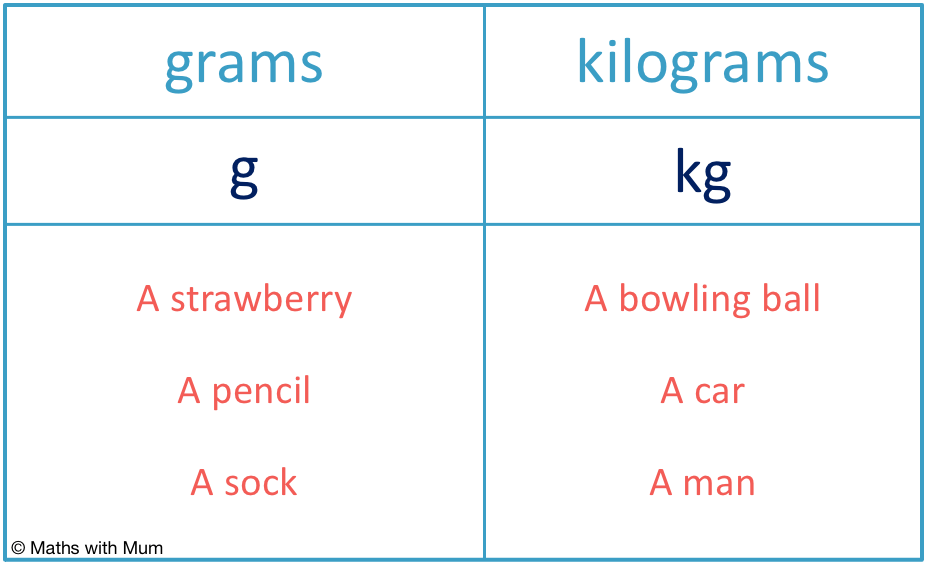• Anything heavier than 1 kilogram is usually weighed in kilograms.
• Anything lighter than 1 kilogram is usually weighed in grams.
• A litre of water weighs 1 kilogram.
• If an item would be heavy to hold in one hand, it is probably best to measure it in kilograms.
• A strawberry, a pencil and a sock can all easily be held in one hand and so, we should measure them in grams.
• A bowling ball, a car and a man are all heavy to hold in one hand and so, we would measure them in kilograms.
• Even though the bowling ball can be held in one hand, it would be hard to do so for long and so, this is a good hint that kilograms might be more appropriate to use.Supporting Lessons# Grams and Kilograms

## How to Decide Whether to Measure Something in Grams or Kilograms?

1 kilogram weighs the same as 1 litre of water. If something weighs 1 kilogram or more, it makes sense to measure it in kilograms. If something is less than a kilogram, it makes sense to measure it in grams. A good rule to follow is if the object is too heavy to hold high in one hand, measure it in kilograms. If the object can be held easily in one hand, measure it in grams.

All objects can be measured in both grams or kilograms but it is best to choose a unit that will not be written as a very small decimal number or a number that is larger than 1000.For example, a strawberry, a pencil and a sock are all objects that can be held easily in one hand and so, they should be measured in grams.

A bowling ball would be heavy to hold up high with one hand and so, kilograms would be a better unit of mass. Both a car and a man are impossible to hold up high with one hand and therefore, kilograms would be the best unit of mass to use.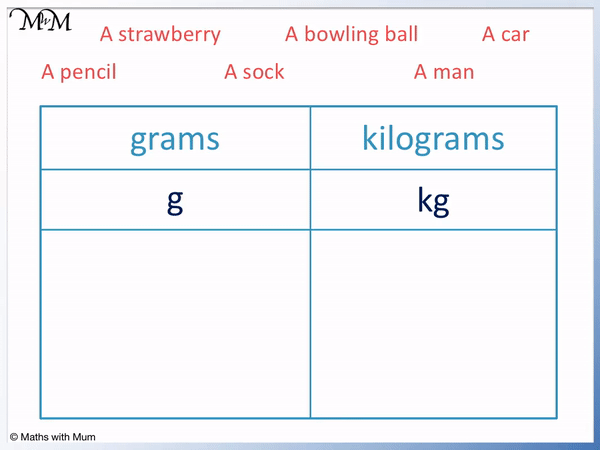It is better to say that a pencil is 10 grams than it is to say that it is 0.01 kilograms. In general, people prefer to use numbers that are not decimals, especially decimals that are less than 0.

It is better to say that a man weighs 80 kilograms than it is to say that a man weighs 80 000 grams. Numbers larger than 1000 are harder to comprehend and compare.

Here is a table showing some examples of objects measured in grams and kilograms.

Object Appropriate Unit of Mass Weight
Pencil grams 10 g
Apple grams 100 g
Watermelon kilograms 10 kg
Mobile phone grams 150 g
Strawberry grams 7 g
An adult man kilograms 80 kg
A cat kilograms 5 kg

## How Heavy is a Gram?

One gram is very light. One gram is as heavy as a blueberry or a paperclip. A pencil weighs about 10 grams, an apple weighs about 100 grams and a loaf of bread weighs about 500 grams.

Each gram can be thought of as being the same weight as a paperclip. So if something weighs 10 grams, it weighs the same as 10 paperclips.Grams are written for short as g. 1 gram is written as 1 g. 10 grams is written as 10 g.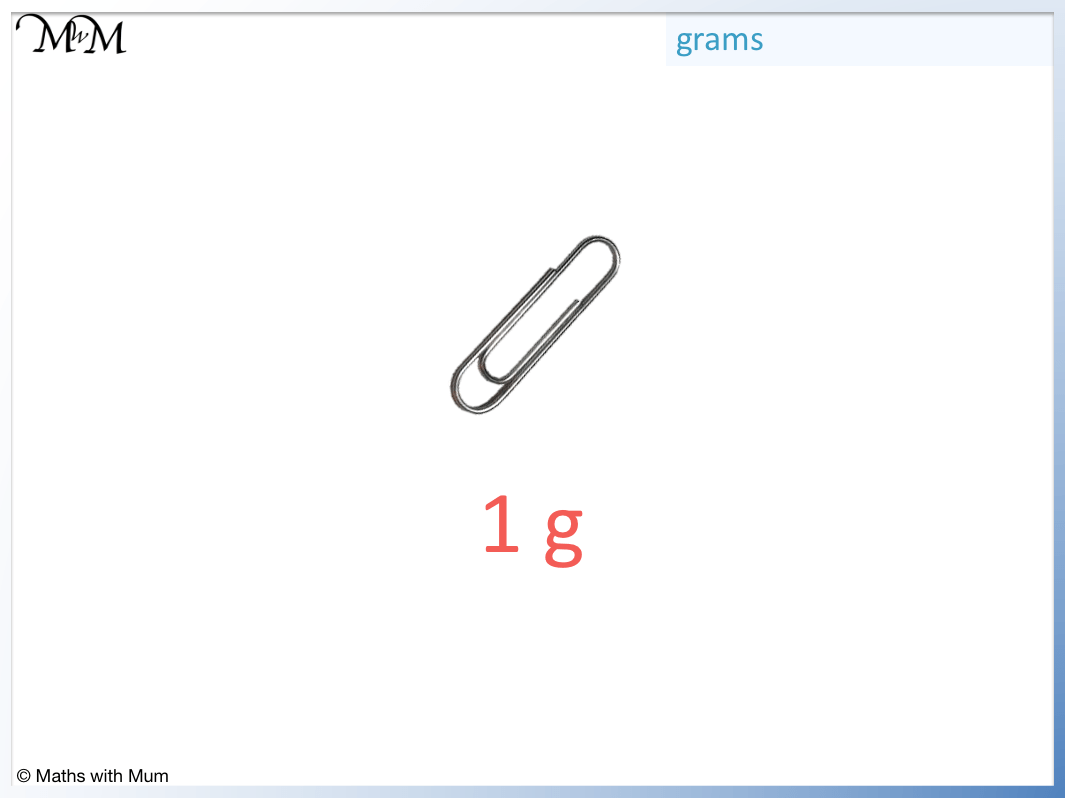A gram is the same the weight as a blueberry.This means that an acorn weighs about the same as 5 paperclips.

An apple weighs about 100 grams.This means that an apple weighs the same as 100 paperclips or 20 acorns.This means that a loaf of bread weighs the same as 500 paperclips or 5 apples.

## How Heavy is One Kilogram?

One kilogram by definition is the weight of 1 litre of water. Some other objects that weigh 1 kilogram are a pineapple, a standard bag of sugar and a toaster. A large family drink bottle weighs 2 kilograms, a house cat weighs 5 kilograms and a large dog weighs about 30 kilograms.

A kilogram is 1000 grams. The suffix of kilo means 1000.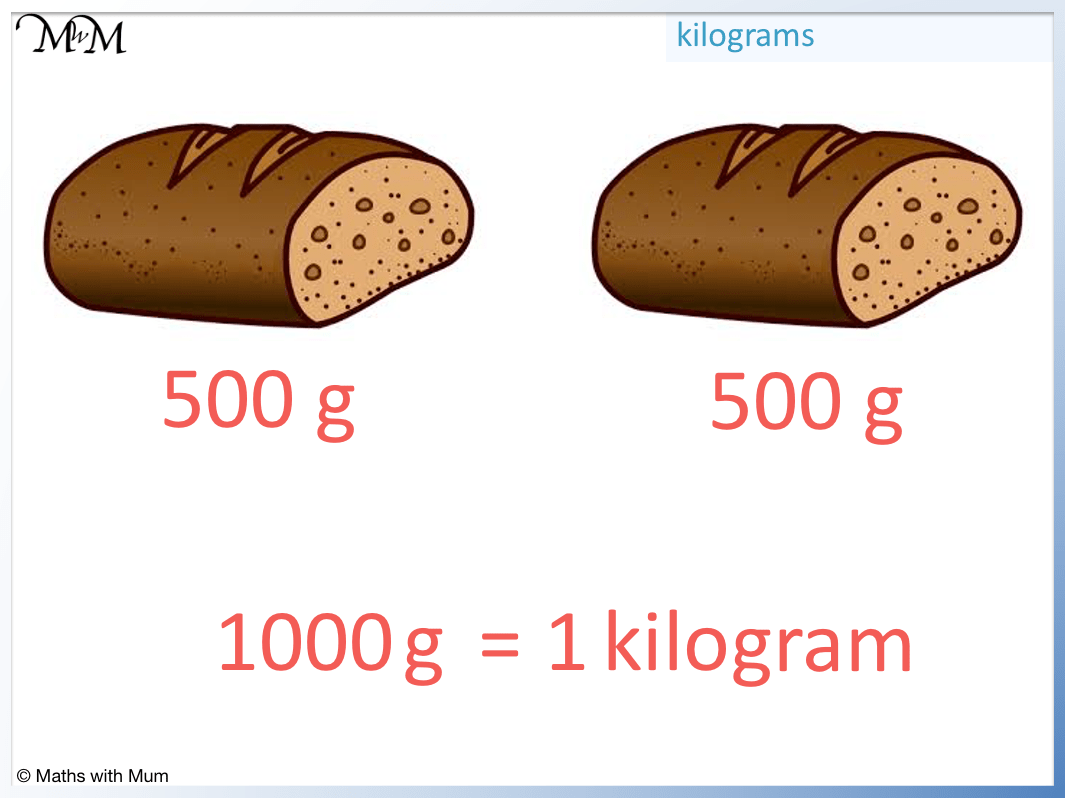If one loaf of bread weighs 500 grams, then two loaves of bread weigh 1 kilogram. This is because 500 + 500 = 1000. 1000 grams is 1 kilogram.

An adult man weighs 80 kg and so, an adult man weighs about the same as 80 one-litre bottles of water.

A car weighs about 1500 kg and so, a car weighs about the same as 20 adult men or 1500 one-litre bottles of water.

## How to Convert from Grams to Kilograms

To convert from grams to kilograms, divide the amount by 1000. This can be done by moving the decimal point 3 places to the left. For example, 375 g = 0.375 kg.Here are some examples of converting from grams to kilograms by dividing by 1000.

Weight in grams Conversion Weight in kilograms
10 000 g ÷ 1000 10 kg
5000 g ÷ 1000 5 kg
3400 g ÷ 1000 3.4 kg
6723 g ÷ 1000 6.723 kg
300 g ÷ 1000 0.3 kg
275 g ÷ 1000 0.275 kg
34 g ÷ 1000 0.034 kg
5 g ÷ 1000 0.005 kg
1.2 g ÷ 1000 0.0012 kg
0.068 g ÷ 1000 0.000068 kg

## How to Convert from Kilograms to Grams

There are 1000 grams in a kilogram, so to convert from kilograms to grams, simply multiply the amount by 1000. For example 5.2 kg = 5200 g.Here are some examples of converting from kilograms to grams by multiplying by 1000.

Weight in kilograms Conversion Weight in grams
325 kg × 1000 325 000 g
201 kg × 1000 201 000 g
7 kg × 1000 7000 g
3.2 kg × 1000 3200 g
0.5 kg × 1000 500 g
0.21 kg × 1000 210 g
0.09 kg × 1000 90 g
0.086 kg × 1000 86 g
0.003 kg × 1000 3 g
0.00064 kg × 1000 0.64 g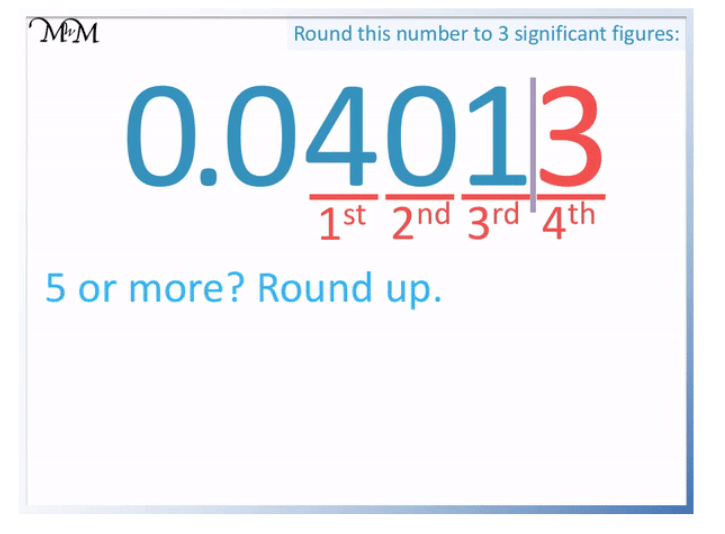Now try our lesson on Metric Units of Volume where we learn about the different units for measuring volume.